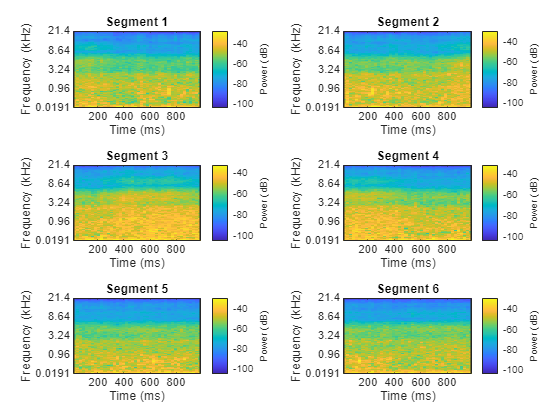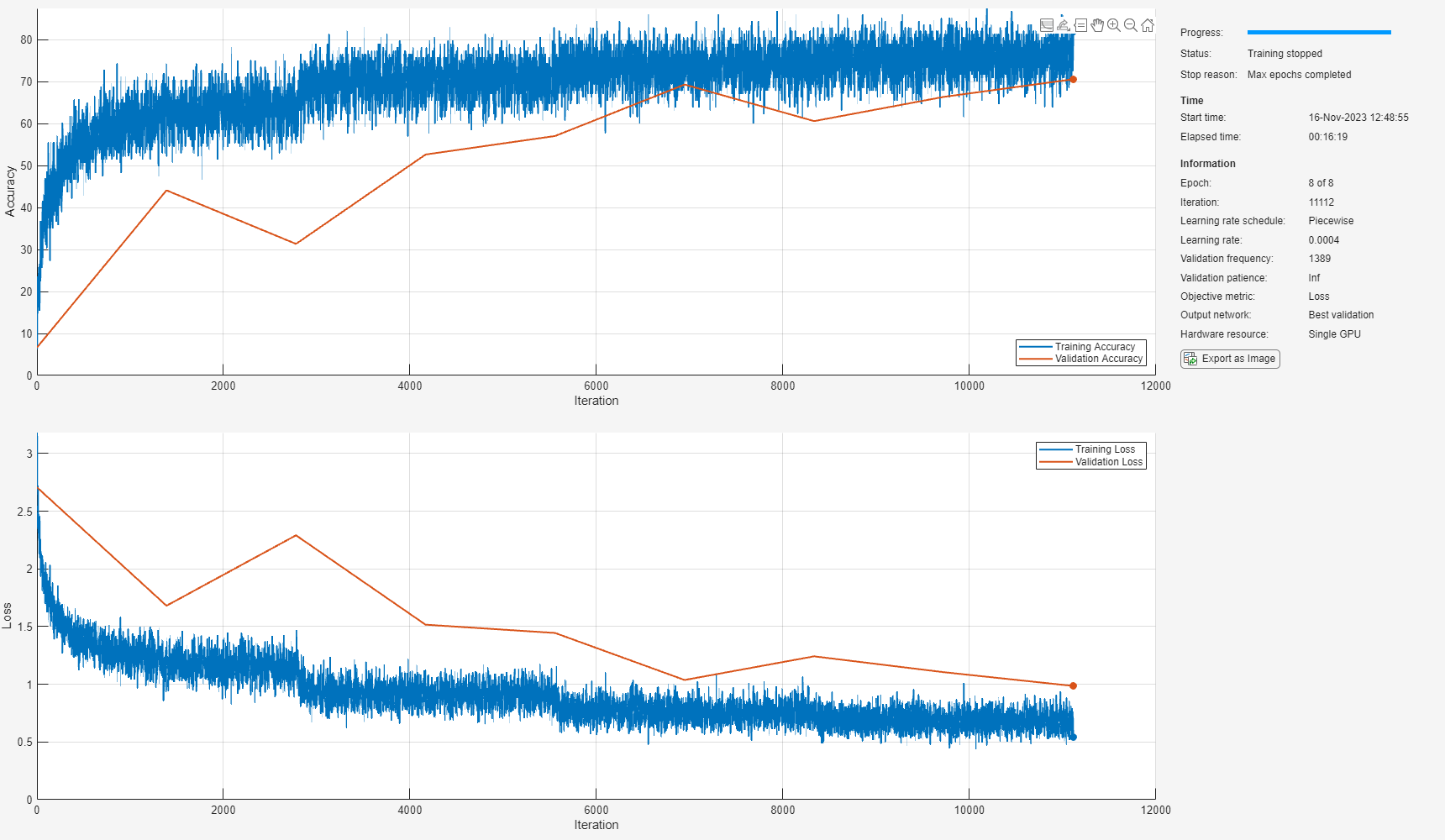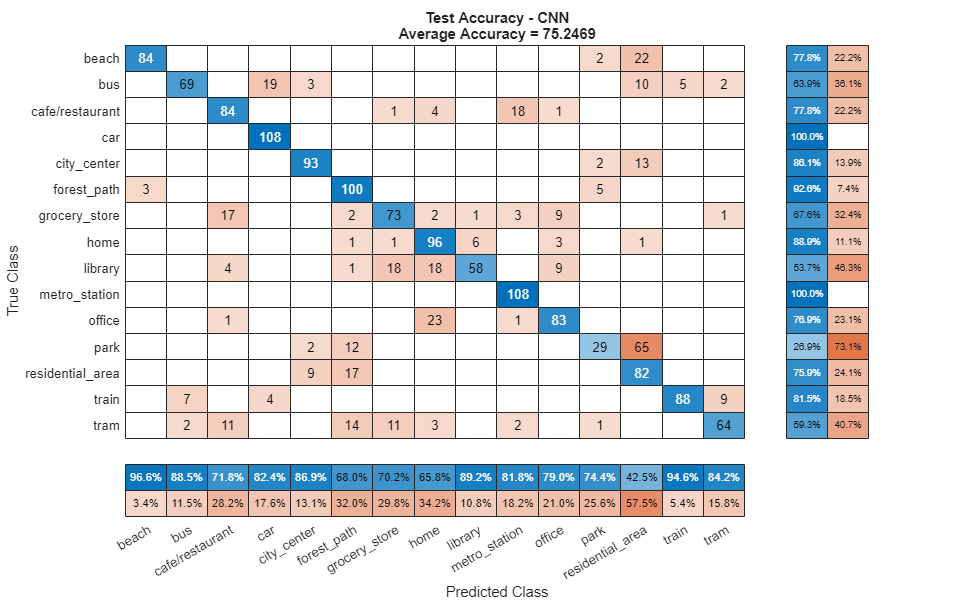# Acoustic Scene Recognition Using Late Fusion

This example shows how to create a multi-model late fusion system for acoustic scene recognition. The example trains a convolutional neural network (CNN) using mel spectrograms and an ensemble classifier using wavelet scattering. The example uses the TUT dataset for training and evaluation .

### Introduction

Acoustic scene classification (ASC) is the task of classifying environments from the sounds they produce. ASC is a generic classification problem that is foundational for context awareness in devices, robots, and many other applications . Early attempts at ASC used mel-frequency cepstral coefficients (`mfcc`) and Gaussian mixture models (GMMs) to describe their statistical distribution. Other popular features used for ASC include zero crossing rate, spectral centroid (`spectralCentroid`), spectral rolloff (`spectralRolloffPoint`), spectral flux (`spectralFlux` ), and linear prediction coefficients (`lpc`) . Hidden Markov models (HMMs) were trained to describe the temporal evolution of the GMMs. More recently, the best performing systems have used deep learning, usually CNNs, and a fusion of multiple models. The most popular feature for top-ranked systems in the DCASE 2017 contest was the mel spectrogram (`melSpectrogram`). The top-ranked systems in the challenge used late fusion and data augmentation to help their systems generalize.

To illustrate a simple approach that produces reasonable results, this example trains a CNN using mel spectrograms and an ensemble classifier using wavelet scattering. The CNN and ensemble classifier produce roughly equivalent overall accuracy, but perform better at distinguishing different acoustic scenes. To increase overall accuracy, you merge the CNN and ensemble classifier results using late fusion.

### Load Acoustic Scene Recognition Data Set

```downloadFolder = tempdir; dataset = fullfile(downloadFolder,"TUT-acoustic-scenes-2017"); if ~datasetExists(dataset) disp("Downloading TUT-acoustic-scenes-2017 (15.5 GB) ...") HelperDownload_TUT_acoustic_scenes_2017(dataset); end```

Read in the development set metadata as a table. Name the table variables `FileName`, `AcousticScene`, and `SpecificLocation`.

```trainMetaData = readtable(fullfile(dataset,"TUT-acoustic-scenes-2017-development","meta"), ... Delimiter={'\t'}, ... ReadVariableNames=false); trainMetaData.Properties.VariableNames = ["FileName","AcousticScene","SpecificLocation"]; head(trainMetaData)```
```ans=8×3 table FileName AcousticScene SpecificLocation __________________________ _____________ ________________ {'audio/b020_90_100.wav' } {'beach'} {'b020'} {'audio/b020_110_120.wav'} {'beach'} {'b020'} {'audio/b020_100_110.wav'} {'beach'} {'b020'} {'audio/b020_40_50.wav' } {'beach'} {'b020'} {'audio/b020_50_60.wav' } {'beach'} {'b020'} {'audio/b020_30_40.wav' } {'beach'} {'b020'} {'audio/b020_160_170.wav'} {'beach'} {'b020'} {'audio/b020_170_180.wav'} {'beach'} {'b020'} ```
```testMetaData = readtable(fullfile(dataset,"TUT-acoustic-scenes-2017-evaluation","meta"), ... Delimiter={'\t'}, ... ReadVariableNames=false); testMetaData.Properties.VariableNames = ["FileName","AcousticScene","SpecificLocation"]; head(testMetaData)```
```ans=8×3 table FileName AcousticScene SpecificLocation __________________ _____________ ________________ {'audio/1245.wav'} {'beach'} {'b174'} {'audio/1456.wav'} {'beach'} {'b174'} {'audio/1318.wav'} {'beach'} {'b174'} {'audio/967.wav' } {'beach'} {'b174'} {'audio/203.wav' } {'beach'} {'b174'} {'audio/777.wav' } {'beach'} {'b174'} {'audio/231.wav' } {'beach'} {'b174'} {'audio/768.wav' } {'beach'} {'b174'} ```

Note that the specific recording locations in the test set do not intersect with the specific recording locations in the development set. This makes it easier to validate that the trained models can generalize to real-world scenarios.

```sharedRecordingLocations = intersect(testMetaData.SpecificLocation,trainMetaData.SpecificLocation); disp("Number of specific recording locations in both train and test sets = " + numel(sharedRecordingLocations))```
```Number of specific recording locations in both train and test sets = 0 ```

The first variable of the metadata tables contains the file names. Concatenate the file names with the file paths.

```trainFilePaths = fullfile(dataset,"TUT-acoustic-scenes-2017-development",trainMetaData.FileName); testFilePaths = fullfile(dataset,"TUT-acoustic-scenes-2017-evaluation",testMetaData.FileName);```

There may be files listed in the metadata that are not present in the data set. Remove the filepaths and acoustic scene labels that correspond to the missing files.

```ads = audioDatastore(dataset,IncludeSubfolders=true); allFiles = ads.Files; trainIdxToRemove = ~ismember(trainFilePaths,allFiles); trainFilePaths(trainIdxToRemove) = []; trainLabels = categorical(trainMetaData.AcousticScene); trainLabels(trainIdxToRemove) = []; testIdxToRemove = ~ismember(testFilePaths,allFiles); testFilePaths(testIdxToRemove) = []; testLabels = categorical(testMetaData.AcousticScene); testLabels(testIdxToRemove) = [];```

Create audio datastores for the train and test sets. Set the `Labels` property of the `audioDatastore` to the acoustic scene. Call `countEachLabel` to verify an even distribution of labels in both the train and test sets.

```adsTrain = audioDatastore(trainFilePaths, ... Labels=trainLabels, ... IncludeSubfolders=true); display(countEachLabel(adsTrain))```
``` 15×2 table Label Count ________________ _____ beach 312 bus 312 cafe/restaurant 312 car 312 city_center 312 forest_path 312 grocery_store 312 home 312 library 312 metro_station 312 office 312 park 312 residential_area 312 train 312 tram 312 ```
```adsTest = audioDatastore(testFilePaths, ... Labels=categorical(testMetaData.AcousticScene), ... IncludeSubfolders=true); display(countEachLabel(adsTest))```
``` 15×2 table Label Count ________________ _____ beach 108 bus 108 cafe/restaurant 108 car 108 city_center 108 forest_path 108 grocery_store 108 home 108 library 108 metro_station 108 office 108 park 108 residential_area 108 train 108 tram 108 ```

You can reduce the data set used in this example to speed up the run time at the cost of performance. In general, reducing the data set is a good practice for development and debugging. Set `speedupExample` to `true` to reduce the data set.

```speedupExample =false; if speedupExample adsTrain = splitEachLabel(adsTrain,20); adsTest = splitEachLabel(adsTest,10); end```

Call `read` to get the data and sample rate of a file from the train set. Audio in the database has consistent sample rate and duration. Normalize the audio and listen to it. Display the corresponding label.

```[data,adsInfo] = read(adsTrain); data = data./max(data,[],"all"); fs = adsInfo.SampleRate; sound(data,fs) disp("Acoustic scene = " + string(adsTrain.Labels(1)))```
```Acoustic scene = beach ```

Call `reset` to return the datastore to its initial condition.

`reset(adsTrain)`

### Feature Extraction for CNN

Each audio clip in the dataset consists of 10 seconds of stereo (left-right) audio. The feature extraction pipeline and the CNN architecture in this example are based on . Hyperparameters for the feature extraction, the CNN architecture, and the training options were modified from the original paper using a systematic hyperparameter optimization workflow.

First, convert the audio to mid-side encoding.  suggests that mid-side encoded data provides better spatial information that the CNN can use to identify moving sources (such as a train moving across an acoustic scene).

`dataMidSide = [sum(data,2),data(:,1)-data(:,2)];`

Divide the signal into one-second segments with overlap. The final system uses a probability-weighted average on the one-second segments to predict the scene for each 10-second audio clip in the test set. Dividing the audio clips into one-second segments makes the network easier to train and helps prevent overfitting to specific acoustic events in the training set. The overlap helps to ensure all combinations of features relative to one another are captured by the training data. It also provides the system with additional data that can be mixed uniquely during augmentation.

```segmentLength = 1; segmentOverlap = 0.5; [dataBufferedMid,~] = buffer(dataMidSide(:,1),round(segmentLength*fs),round(segmentOverlap*fs),"nodelay"); [dataBufferedSide,~] = buffer(dataMidSide(:,2),round(segmentLength*fs),round(segmentOverlap*fs),"nodelay"); dataBuffered = zeros(size(dataBufferedMid,1),size(dataBufferedMid,2)+size(dataBufferedSide,2)); dataBuffered(:,1:2:end) = dataBufferedMid; dataBuffered(:,2:2:end) = dataBufferedSide;```

Use `melSpectrogram` to transform the data into a compact frequency-domain representation. Define parameters for the mel spectrogram as suggested by .

```windowLength = 2048; samplesPerHop = 1024; samplesOverlap = windowLength - samplesPerHop; fftLength = 2*windowLength; numBands = 128;```

`melSpectrogram` operates along channels independently. To optimize processing time, call `melSpectrogram` with the entire buffered signal.

```spec = melSpectrogram(dataBuffered,fs, ... Window=hamming(windowLength,"periodic"), ... OverlapLength=samplesOverlap, ... FFTLength=fftLength, ... NumBands=numBands);```

Convert the mel spectrogram into the logarithmic scale.

`spec = log10(spec+eps);`

Reshape the array to dimensions (Number of bands)-by-(Number of hops)-by-(Number of channels)-by-(Number of segments). When you feed an image into a neural network, the first two dimensions are the height and width of the image, the third dimension is the channels, and the fourth dimension separates the individual images.

`X = reshape(spec,size(spec,1),size(spec,2),size(data,2),[]);`

Call `melSpectrogram` without output arguments to plot the mel spectrogram of the mid channel for the first six of the one-second increments.

```tiledlayout(3,2) for channel = 1:2:11 nexttile melSpectrogram(dataBuffered(:,channel),fs, ... Window=hamming(windowLength,"periodic"), ... OverlapLength=samplesOverlap, ... FFTLength=fftLength, ... NumBands=numBands); title("Segment " + ceil(channel/2)) end```The helper function `HelperSegmentedMelSpectrograms` performs the feature extraction steps outlined above.

To speed up processing, extract mel spectrograms of all audio files in the datastores using `tall` arrays. Unlike in-memory arrays, tall arrays remain unevaluated until you request that the calculations be performed using the `gather` function. This deferred evaluation enables you to work quickly with large data sets. When you eventually request the output using `gather`, MATLAB combines the queued calculations where possible and takes the minimum number of passes through the data. If you have Parallel Computing Toolbox™, you can use tall arrays in your local MATLAB session, or on a local parallel pool. You can also run tall array calculations on a cluster if you have MATLAB® Parallel Server™ installed.

If you do not have Parallel Computing Toolbox™, the code in this example still runs.

```train_set_tall = tall(adsTrain); xTrain = cellfun(@(x)HelperSegmentedMelSpectrograms(x,fs, ... SegmentLength=segmentLength, ... SegmentOverlap=segmentOverlap, ... WindowLength=windowLength, ... HopLength=samplesPerHop, ... NumBands=numBands, ... FFTLength=fftLength), ... train_set_tall, ... UniformOutput=false); xTrain = gather(xTrain);```
```Evaluating tall expression using the Parallel Pool 'local': - Pass 1 of 1: 0% complete Evaluation 0% complete - Pass 1 of 1: Completed in 3 min 56 sec Evaluation completed in 3 min 56 sec ```
```xTrain = cat(4,xTrain{:}); test_set_tall = tall(adsTest); xTest = cellfun(@(x)HelperSegmentedMelSpectrograms(x,fs, ... SegmentLength=segmentLength, ... SegmentOverlap=segmentOverlap, ... WindowLength=windowLength, ... HopLength=samplesPerHop, ... NumBands=numBands, ... FFTLength=fftLength), ... test_set_tall, ... UniformOutput=false); xTest = gather(xTest);```
```Evaluating tall expression using the Parallel Pool 'local': - Pass 1 of 1: Completed in 1 min 26 sec Evaluation completed in 1 min 26 sec ```
`xTest = cat(4,xTest{:});`

Replicate the labels of the training and test sets so that they are in one-to-one correspondence with the segments.

```numSegmentsPer10seconds = size(dataBuffered,2)/2; yTrain = repmat(adsTrain.Labels,1,numSegmentsPer10seconds)'; yTrain = yTrain(:); yTest = repmat(adsTest.Labels,1,numSegmentsPer10seconds)'; yTest = yTest(:);```

### Data Augmentation for CNN

The DCASE 2017 dataset contains a relatively small number of acoustic recordings for the task, and the development set and evaluation set were recorded at different specific locations. As a result, it is easy to overfit to the data during training. One popular method to reduce overfitting is mixup. In mixup, you augment your dataset by mixing the features of two different classes. When you mix the features, you mix the labels in equal proportion. That is:

`$\begin{array}{l}\underset{}{\overset{\sim }{x}}=\lambda {x}_{i}+\left(1-\lambda \right){x}_{j}\\ \underset{}{\overset{\sim }{y}}=\lambda {y}_{i}+\left(1-\lambda \right){y}_{j}\end{array}$`

Mixup was reformulated by  as labels drawn from a probability distribution instead of mixed labels. The implementation of mixup in this example is a simplified version of mixup: each spectrogram is mixed with a spectrogram of a different label with lambda set to 0.5. The original and mixed datasets are combined for training.

```xTrainExtra = xTrain; yTrainExtra = yTrain; lambda = 0.5; for ii = 1:size(xTrain,4) % Find all available spectrograms with different labels. availableSpectrograms = find(yTrain~=yTrain(ii)); % Randomly choose one of the available spectrograms with a different label. numAvailableSpectrograms = numel(availableSpectrograms); idx = randi([1,numAvailableSpectrograms]); % Mix. xTrainExtra(:,:,:,ii) = lambda*xTrain(:,:,:,ii) + (1-lambda)*xTrain(:,:,:,availableSpectrograms(idx)); % Specify the label as randomly set by lambda. if rand > lambda yTrainExtra(ii) = yTrain(availableSpectrograms(idx)); end end xTrain = cat(4,xTrain,xTrainExtra); yTrain = [yTrain;yTrainExtra];```

Call `summary` to display the distribution of labels for the augmented training set.

`summary(yTrain)`
``` beach 11769 bus 11904 cafe/restaurant 11873 car 11820 city_center 11886 forest_path 11936 grocery_store 11914 home 11923 library 11817 metro_station 11804 office 11922 park 11871 residential_area 11704 train 11773 tram 11924 ```

### Define and Train CNN

Define the CNN architecture. This architecture is based on  and modified through trial and error. See List of Deep Learning Layers (Deep Learning Toolbox) to learn more about deep learning layers available in MATLAB®.

```imgSize = [size(xTrain,1),size(xTrain,2),size(xTrain,3)]; numF = 32; layers = [ ... imageInputLayer(imgSize) batchNormalizationLayer convolution2dLayer(3,numF,Padding="same") batchNormalizationLayer reluLayer convolution2dLayer(3,numF,Padding="same") batchNormalizationLayer reluLayer maxPooling2dLayer(3,Stride=2,Padding="same") convolution2dLayer(3,2*numF,Padding="same") batchNormalizationLayer reluLayer convolution2dLayer(3,2*numF,Padding="same") batchNormalizationLayer reluLayer maxPooling2dLayer(3,Stride=2,Padding="same") convolution2dLayer(3,4*numF,Padding="same") batchNormalizationLayer reluLayer convolution2dLayer(3,4*numF,Padding="same") batchNormalizationLayer reluLayer maxPooling2dLayer(3,Stride=2,Padding="same") convolution2dLayer(3,8*numF,Padding="same") batchNormalizationLayer reluLayer convolution2dLayer(3,8*numF,Padding="same") batchNormalizationLayer reluLayer globalAveragePooling2dLayer dropoutLayer(0.5) fullyConnectedLayer(15) softmaxLayer classificationLayer];```

Define `trainingOptions` (Deep Learning Toolbox) for the CNN. These options are based on  and modified through a systematic hyperparameter optimization workflow.

```miniBatchSize = 128; tuneme = 128; lr = 0.05*miniBatchSize/tuneme; options = trainingOptions( ... "sgdm", ... Momentum=0.9, ... L2Regularization=0.005, ... ... MiniBatchSize=miniBatchSize, ... MaxEpochs=8, ... Shuffle="every-epoch", ... ... Plots="training-progress", ... Verbose=false, ... ... InitialLearnRate=lr, ... LearnRateSchedule="piecewise", ... LearnRateDropPeriod=2, ... LearnRateDropFactor=0.2, ... ... ValidationData={xTest,yTest}, ... ValidationFrequency=floor(size(xTrain,4)/miniBatchSize));```

Call `trainNetwork` (Deep Learning Toolbox) to train the network.

`trainedNet = trainNetwork(xTrain,yTrain,layers,options);`### Evaluate CNN

Call `predict` (Deep Learning Toolbox) to predict responses from the trained network using the held-out test set.

`cnnResponsesPerSegment = predict(trainedNet,xTest);`

Average the responses over each 10-second audio clip.

```classes = trainedNet.Layers(end).Classes; numFiles = numel(adsTest.Files); counter = 1; cnnResponses = zeros(numFiles,numel(classes)); for channel = 1:numFiles cnnResponses(channel,:) = sum(cnnResponsesPerSegment(counter:counter+numSegmentsPer10seconds-1,:),1)/numSegmentsPer10seconds; counter = counter + numSegmentsPer10seconds; end```

For each 10-second audio clip, choose the maximum of the predictions, then map it to the corresponding predicted location.

```[~,classIdx] = max(cnnResponses,[],2); cnnPredictedLabels = classes(classIdx);```

Call `confusionchart` (Deep Learning Toolbox) to visualize the accuracy on the test set.

```figure(Units="normalized",Position=[0.2 0.2 0.5 0.5]) confusionchart(adsTest.Labels,cnnPredictedLabels, ... title=["Test Accuracy - CNN","Average Accuracy = " + mean(adsTest.Labels==cnnPredictedLabels)*100], ... ColumnSummary="column-normalized",RowSummary="row-normalized");```### Feature Extraction for Ensemble Classifier

Wavelet scattering has been shown in  to provide a good representation of acoustic scenes. Define a `waveletScattering` (Wavelet Toolbox) object. The invariance scale and quality factors were determined through trial and error.

```sf = waveletScattering(SignalLength=size(data,1), ... SamplingFrequency=fs, ... InvarianceScale=0.75, ... QualityFactors=[4 1]);```

Convert the audio signal to mono, and then call `featureMatrix` (Wavelet Toolbox) to return the scattering coefficients for the scattering decomposition framework, `sf`.

```dataMono = mean(data,2); scatteringCoeffients = featureMatrix(sf,dataMono,Transform="log");```

Average the scattering coefficients over the 10-second audio clip.

```featureVector = mean(scatteringCoeffients,2); disp("Number of wavelet features per 10-second clip = " + numel(featureVector));```
```Number of wavelet features per 10-second clip = 286 ```

The helper function `HelperWaveletFeatureVector` performs the above steps. Use a `tall` array with `cellfun` and `HelperWaveletFeatureVector` to parallelize the feature extraction. Extract wavelet feature vectors for the train and test sets.

```scatteringTrain = cellfun(@(x)HelperWaveletFeatureVector(x,sf),train_set_tall,UniformOutput=false); xTrain = gather(scatteringTrain); xTrain = cell2mat(xTrain')';```
```scatteringTest = cellfun(@(x)HelperWaveletFeatureVector(x,sf),test_set_tall,UniformOutput=false); xTest = gather(scatteringTest); xTest = cell2mat(xTest')';```

### Define and Train Ensemble Classifier

Use `fitcensemble` to create a trained classification ensemble model (`ClassificationEnsemble`).

```subspaceDimension = min(150,size(xTrain,2) - 1); numLearningCycles = 30; classificationEnsemble = fitcensemble(xTrain,adsTrain.Labels, ... Method="Subspace", ... NumLearningCycles=numLearningCycles, ... Learners="discriminant", ... NPredToSample=subspaceDimension, ... ClassNames=removecats(unique(adsTrain.Labels)));```

### Evaluate Ensemble Classifier

For each 10-second audio clip, call `predict` to return the labels and the weights, then map it to the corresponding predicted location. Call `confusionchart` (Deep Learning Toolbox) to visualize the accuracy on the test set.

```[waveletPredictedLabels,waveletResponses] = predict(classificationEnsemble,xTest); figure(Units="normalized",Position=[0.2 0.2 0.5 0.5]) confusionchart(adsTest.Labels,waveletPredictedLabels, ... title=["Test Accuracy - Wavelet Scattering","Average Accuracy = " + mean(adsTest.Labels==waveletPredictedLabels)*100], ... ColumnSummary="column-normalized",RowSummary="row-normalized");````fprintf('Average accuracy of classifier = %0.2f\n',mean(adsTest.Labels==waveletPredictedLabels)*100)`
```Average accuracy of classifier = 75.74 ```

### Apply Late Fusion

For each 10-second clip, calling `predict` on the wavelet classifier and the CNN returns a vector indicating the relative confidence in their decision. Multiply the `waveletResponses` with the `cnnResponses` to create a late fusion system.

```fused = waveletResponses.*cnnResponses; [~,classIdx] = max(fused,[],2); predictedLabels = classes(classIdx);```

### Evaluate Late Fusion

Call `confusionchart` to visualize the fused classification accuracy.

```figure(Units="normalized",Position=[0.2 0.2 0.5 0.5]) confusionchart(adsTest.Labels,predictedLabels, ... Title=["Test Accuracy - Fusion","Average Accuracy = " + mean(adsTest.Labels==predictedLabels)*100], ... ColumnSummary="column-normalized",RowSummary="row-normalized");```### Supporting Functions

#### HelperSegmentedMelSpectrograms

```function X = HelperSegmentedMelSpectrograms(x,fs,varargin) % Copyright 2019-2021 The MathWorks, Inc. p = inputParser; addParameter(p,WindowLength=1024); addParameter(p,HopLength=512); addParameter(p,NumBands=128); addParameter(p,SegmentLength=1); addParameter(p,SegmentOverlap=0); addParameter(p,FFTLength=1024); parse(p,varargin{:}) params = p.Results; x = [sum(x,2),x(:,1)-x(:,2)]; x = x./max(max(x)); [xb_m,~] = buffer(x(:,1),round(params.SegmentLength*fs),round(params.SegmentOverlap*fs),"nodelay"); [xb_s,~] = buffer(x(:,2),round(params.SegmentLength*fs),round(params.SegmentOverlap*fs),"nodelay"); xb = zeros(size(xb_m,1),size(xb_m,2)+size(xb_s,2)); xb(:,1:2:end) = xb_m; xb(:,2:2:end) = xb_s; spec = melSpectrogram(xb,fs, ... Window=hamming(params.WindowLength,"periodic"), ... OverlapLength=params.WindowLength - params.HopLength, ... FFTLength=params.FFTLength, ... NumBands=params.NumBands, ... FrequencyRange=[0,floor(fs/2)]); spec = log10(spec+eps); X = reshape(spec,size(spec,1),size(spec,2),size(x,2),[]); end```

#### HelperWaveletFeatureExtractor

```function features = HelperWaveletFeatureVector(x,sf) % Copyright 2019-2021 The MathWorks, Inc. x = mean(x,2); features = featureMatrix(sf,x,Transform="log"); features = mean(features,2); end```

### References

 A. Mesaros, T. Heittola, and T. Virtanen. Acoustic Scene Classification: an Overview of DCASE 2017 Challenge Entries. In proc. International Workshop on Acoustic Signal Enhancement, 2018.

 Huszar, Ferenc. "Mixup: Data-Dependent Data Augmentation." InFERENCe. November 03, 2017. Accessed January 15, 2019. https://www.inference.vc/mixup-data-dependent-data-augmentation/.

 Han, Yoonchang, Jeongsoo Park, and Kyogu Lee. "Convolutional neural networks with binaural representations and background subtraction for acoustic scene classification." the Detection and Classification of Acoustic Scenes and Events (DCASE) (2017): 1-5.

 Lostanlen, Vincent, and Joakim Anden. Binaural scene classification with wavelet scattering. Technical Report, DCASE2016 Challenge, 2016.

 A. J. Eronen, V. T. Peltonen, J. T. Tuomi, A. P. Klapuri, S. Fagerlund, T. Sorsa, G. Lorho, and J. Huopaniemi, "Audio-based context recognition," IEEE Trans. on Audio, Speech, and Language Processing, vol 14, no. 1, pp. 321-329, Jan 2006.